Name: ___________________Date:___________________

kwizNET Subscribers, please login to turn off the Ads!
 Email us to get an instant 20% discount on highly effective K-12 Math & English kwizNET Programs!

### Grade 7 - Mathematics9.38 Finding Area of Polygons

 Example 1: Find the area of the figure.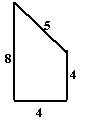The figure can be divided into a square and a right angle triangle.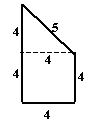Area = Area of square + Area of right angle triangle Area of the square = 4 x 4 = 16 cm2 Area of right angle triangle = 1/2 x 4 x 4 = 8cm2 Area of the figure = 16 + 8 = 24 cm2 Example 2: Find the area of the figure.The figure can be divided into 4 rectangles and 1 square.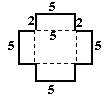Area = Area of 4 rectangles + Area of square Area of one rectangle = 5 x 2 = 10 cm2 Area of 4 rectangles = 4 x 10 = 40 cm2 Area of square = 5 x 5 = 25 cm2 Area = 40 + 25 = 65 cm2 Example 3: Find the area of the figure.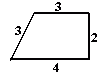The figure can be divided into parallelogram and a right angle triangle.Area = Area of parallelogram + Area of right angle triangle Area of parallelogram = 3 x 3 = 9 cm2 Area of right angle triangle = 1/2 x 1 x 2 = 1 cm2 Area = 9 + 1 = 10 cm2 Example 4: Find the area of the figure.The figure can be divided into two rectangles.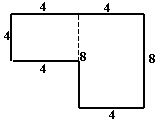Area = Area of square + Area of rectangle Area of square = 4 x 4 = 16 cm2 Area of rectangle = 8 x 4 = 32 cm2 Area = 16 + 32 = 48 cm2 Area = 9 + 1 = 10 cm2 Directions: Find the area of the figure.Name: ___________________Date:___________________

### Grade 7 - Mathematics9.38 Finding Area of Polygons

 Q 1: Find the area of the figure.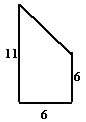Answer: Q 2: Find the area of the figure.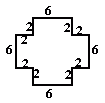Answer: Question 3: This question is available to subscribers only! Question 4: This question is available to subscribers only!

#### Subscription to kwizNET Learning System costs less than \$1 per month & offers the following benefits:

• Unrestricted access to grade appropriate lessons, quizzes, & printable worksheets
• Instant scoring of online quizzes
• Progress tracking and award certificates to keep your student motivated
• Unlimited practice with auto-generated 'WIZ MATH' quizzes
• Child-friendly website with no advertisements

© 2003-2007 kwizNET Learning System LLC. All rights reserved. This material may not be reproduced, displayed, modified or distributed without the express prior written permission of the copyright holder. For permission, contact info@kwizNET.com
For unlimited printable worksheets & more, go to http://www.kwizNET.com.